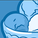# QlikView App Dev

Discussion Board for collaboration related to QlikView App Development.

Announcements
Support Case Portal will move to Qlik Community Oct 4. Temporary case outage Oct 3. READ DETAILS
cancel
Showing results for
Did you mean:Contributor III

## Rolling Sum on Dimension by Specific Timeframe

Hello,

I am trying to calculate a rolling sum, by a dimension called YYYYWK.

The YYYYWK is a format that follows 201801 - 201852, or 201901 - 201952, for example. It is every week for a year.

What I am trying to to do is, at a product level, calculate its sum for the past 13 weeks. Now I know I could do a rolling 13wk sum, but the issue is I do not want it to go back and calculate the previous 13 entries, rather the previous 13 weeks, even if some of the weeks do not have a weekly sum associated to it.

For example, please see excel screenshot below. In the last entry, where YYYYWK = 201902, the rolled sum should be equal to 7938. It should be taking the sums from weeks that are equal to, or 13 weeks less than 201902. (201902+201901+201852+201851+201850+201849+201848+201847+201846+201845+201844+201843+201842).

Again, what it should not be doing, is taking the sum of 201902, and the 12 entries that come before it.

Here is the formula that I did try (where \$(cWeek-13Weeks) is a variable pointing to the week 13weeks from week selected:

=sum({<YYYYWK = {">=\$(cWeek-13Weeks)"}>} Sum)

That does not, however, work. I have also tried putting this formula in with the range sum function, and even using the aggr function to see if that would help, but of course it did not.

Any help on how to write something that can take a rolling sum by specific dates would be very helpful.

Regards

Labels (4)

• ### SumofValue

0 RepliesCommunity Browser